﻿ 焊接残余应力对环肋圆柱壳声辐射特性影响分析
 舰船科学技术2019, Vol. 41Issue (6): 9-14PDF

1. 上海交通大学 海洋工程国家重点实验室，上海 200240;
2. 江南造船（集团）有限责任公司，上海 201913

Research on the influence of welding residual stress on acoustic radiation characteristics of ring-stiffened cylindrical shell
GUO Yong-jin1, KONG Ling-qing2, CHEN Lu-yun1, WANG Hong-dong1
1. State Key Laboratory of Ocean Engineering, Shanghai Jiao Tong University, Shanghai 200240, China;
2. Jiangnan Shipyard(Group) Co.,Ltd., Shanghai 201913, China
Abstract: The ring-stiffened cylindrical shell was taken to research the influence of welding residual stress on the structural acoustic radiation characteristics. The structural-acoustic equation of cylindrical shell with pre-stress was proposed. The structural model was established to conduct the numerical analysis. The residual stress was applied as a variable to research the influence of residual stress amplitude and weld type on structural acoustic radiation power and acoustic radiation directivity. The results show that due to the welding residual stress, the stiffness of the structure is changed, then the structural acoustic radiation characteristics is influenced. The extent of influence is related to the stress amplitude and weld type. The research can provide a reference for the accurate prediction of structural acoustic radiation characteristics.
Key words: ring-stiffened cylindrical shell     welding residual stress     acoustic radiation power     acoustic radiation directivity
0 引　言

1 含预应力的圆柱壳振动-声辐射方程

 ${u} = {[{u_x}\;\;{u_\theta }\;\;{u_r}]^{{\rm T}}}{\text{。}}$ (1)

 $\int_{{{\varOmega }_{s}}} {\delta { \varepsilon }^{{\rm T}}} {{D\varepsilon }}{{\rm d}}{\varOmega _{s}} + \int_{{{\varOmega }_{s}}} {\delta {{u}^{{\rm T}}}} \rho {{{\ddot u}\varepsilon }}{{\rm d}}{\varOmega _{s}} - \int_{{{\varOmega }_{s}}} {\delta {{u}^{{\rm T}}}} {{F}}{{\rm d}}{\varOmega _{s}}{{ = }}0{\text{。}}$ (2)

 ${{u}}(x,\theta ,r,t) = {[{{N}_{{s}}}{\bar{ u}_x}\;\;{{N}_{{s}}}{\bar{ u}_\theta }\;\;{{N}_{{s}}}{\bar{ u}_r}]^{{\rm T}}} = { N}{\bar{ u}}{\text{，}}$ (3)

 ${{N}}{{ = }}\left[ {\begin{array}{*{20}{c}} {{{N}_{{s}}}}&{{0}}&{{0}} \\ {{0}}&{{{N}_{{s}}}}&{{0}} \\ {{0}}&{{0}}&{{{N}_{{s}}}} \end{array}} \right]{\text{，}}$

 ${{m}_s}{\bar{\ddot { u}}} + {{k}_{{s}}}{\bar{ u}}+ { F} =0{\text{。}}$ (4)

 ${{\nabla }^2}{p}(x,\theta ,r,t) + {k^2}{p}(x,\theta ,r,t) = 0{\text{，}}$ (5)
 ${\left. {\frac{{{\partial ^2}{{u}_r}}}{{\partial {t^2}}} = - \frac{1}{{{\rho _f}}}\frac{{\partial {p}(x,\theta ,r,t)}}{{\partial {r}}}} \right|_{r = R}}{\text{。}}\hspace{15pt}$ (6)

 $P = \frac{1}{2}{Re} \int_{{\varOmega _s}} {{{p}_f}} {v}_n^*{{\rm d}}{\varOmega _s}{\text{。}}$ (7)

 $P = \frac{1}{2}{Re} \sum\limits_{j = 1}^{{N_e}} {\int_{{\varOmega _{s,e}}} {{{p}_{f,j}}} {v}_{n,j}^*{{\rm d}}{\varOmega _s}}{\text{。}}$ (8)

 ${P_j} = \frac{1}{2}{Re} \int_{{\varOmega _{s,e}}} {{{p}_j}^{{\rm T}}} {N}{{N}^{{\rm T}}}{v}_{n,j}^*{{\rm d}}{\varOmega _{s,j}}{\text{。}}$ (9)

2 计算模型 2.1 模型基本参数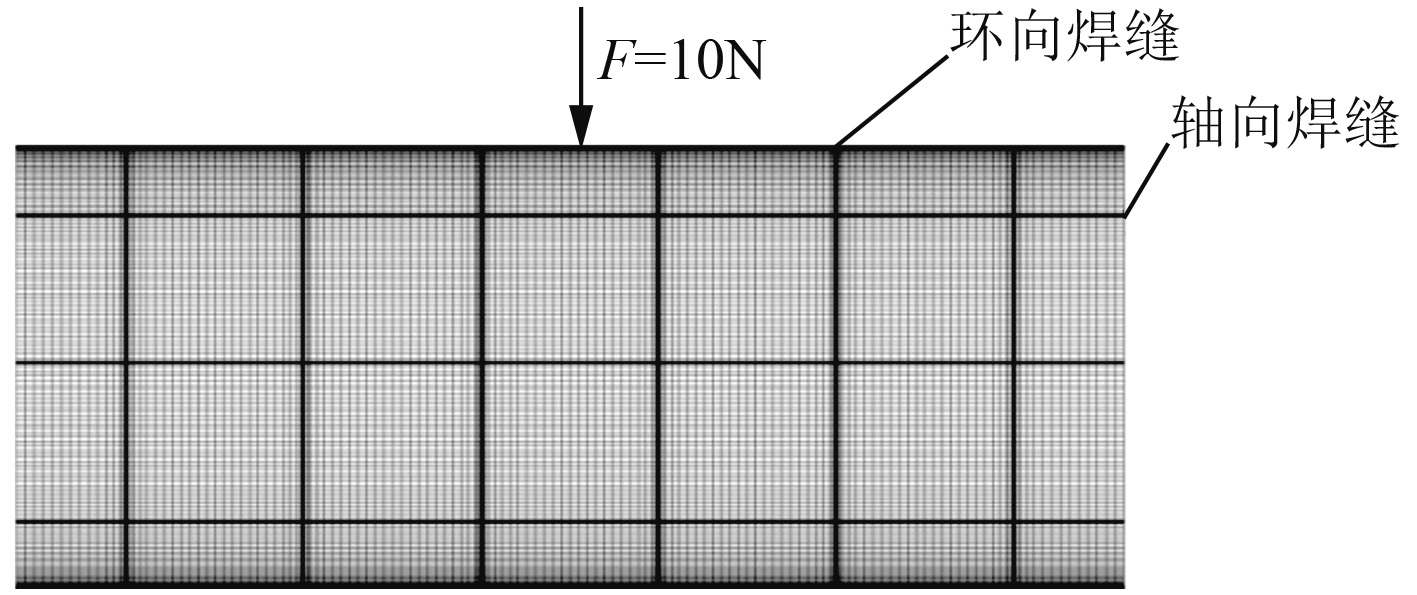图 1 环肋圆柱壳模型 Fig. 1 Model of ring-stiffened cylindrical shell
2.2 焊接残余应力大小及分布表 1 环向焊缝残余应力分布 Tab.1 The distribution of welding residual stress of circumferential weld表 2 轴向焊缝残余应力分布 Tab.2 The distribution of welding residual stress of axial weld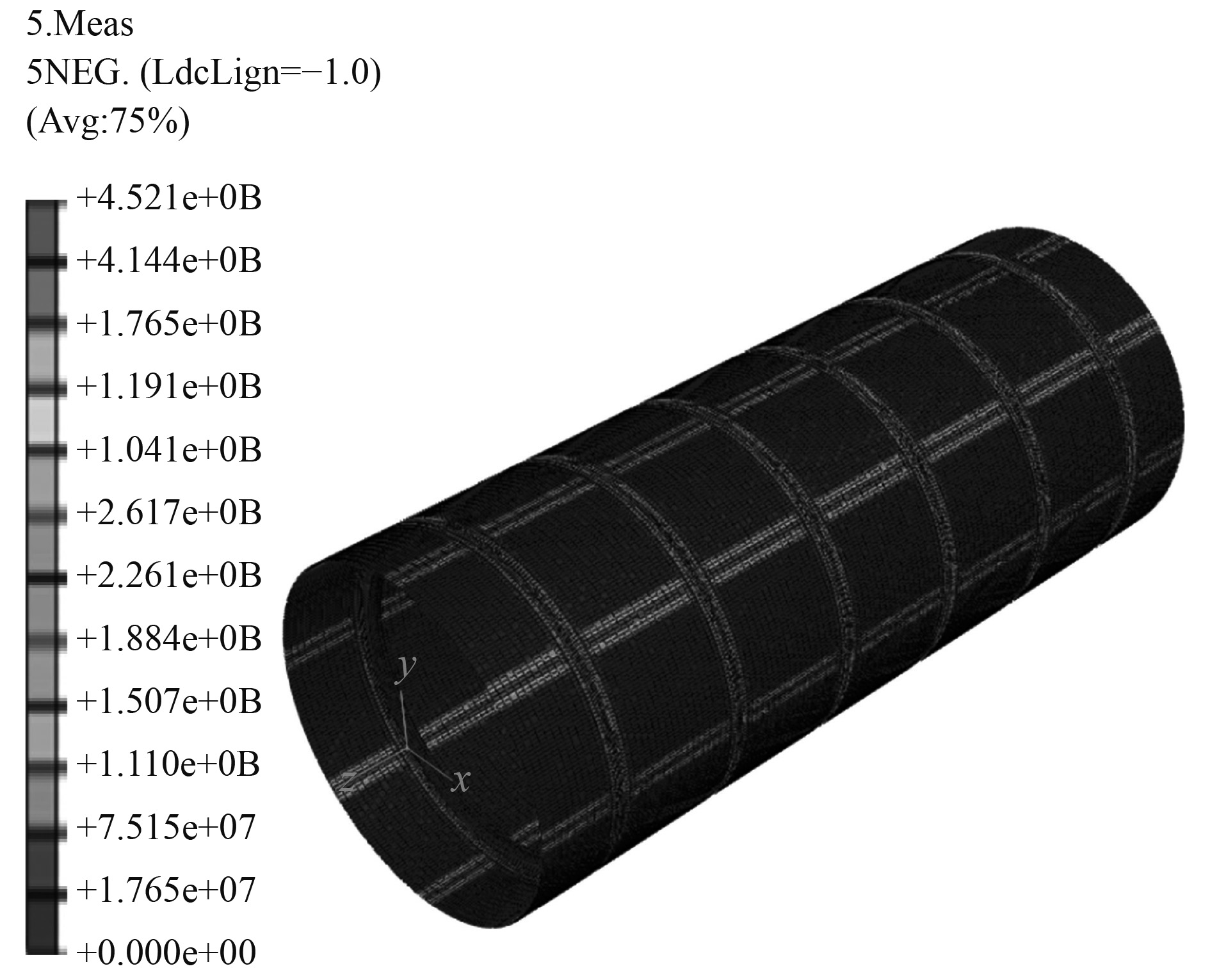图 2 含焊接残余应力有限元模型 Fig. 2 Finite element model with welding residual stress
3 数值计算结果与分析

3.1 含焊接残余应力的结构声辐射变化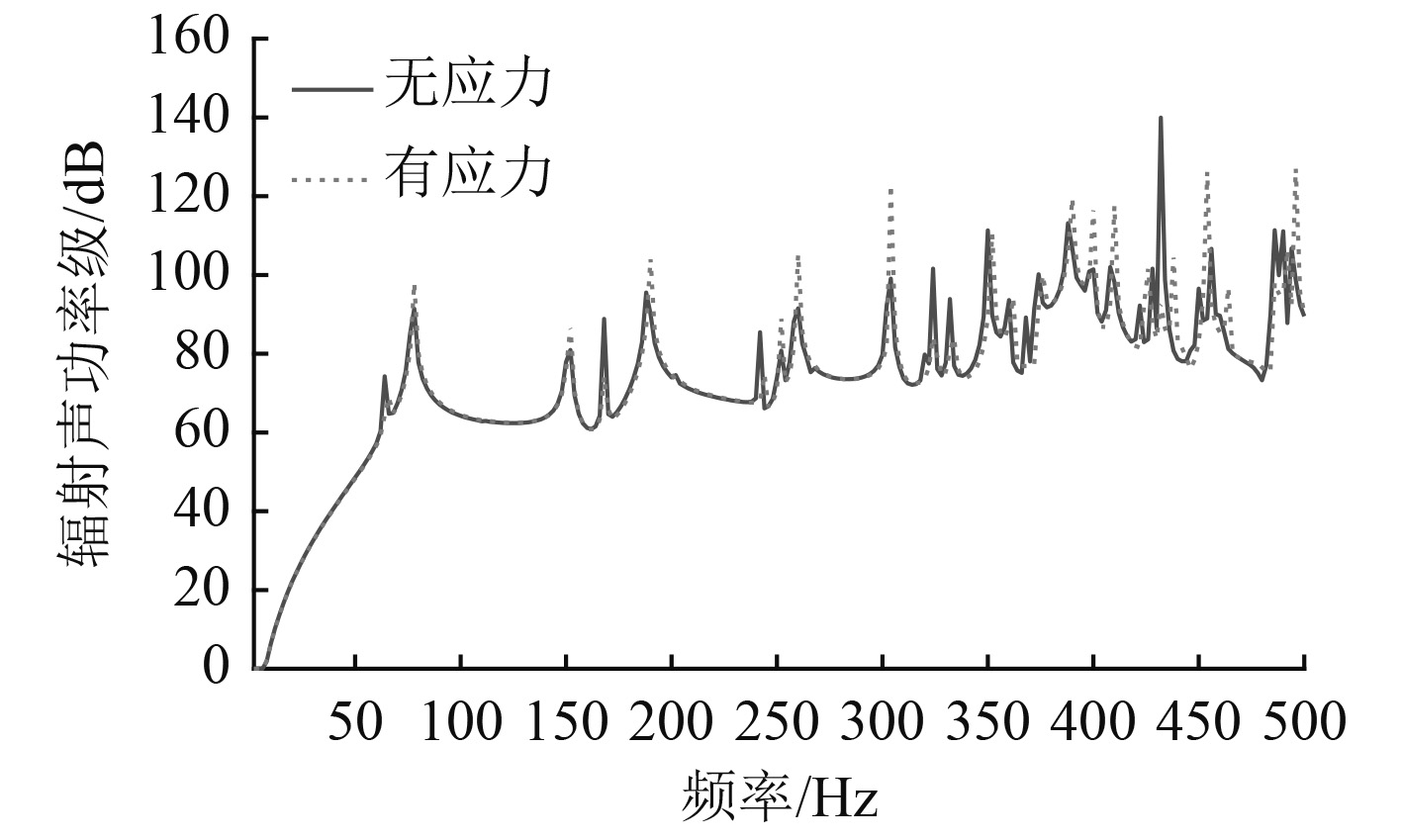图 3 结构声辐射功率对比 Fig. 3 Comparison of structural acoustic radiation power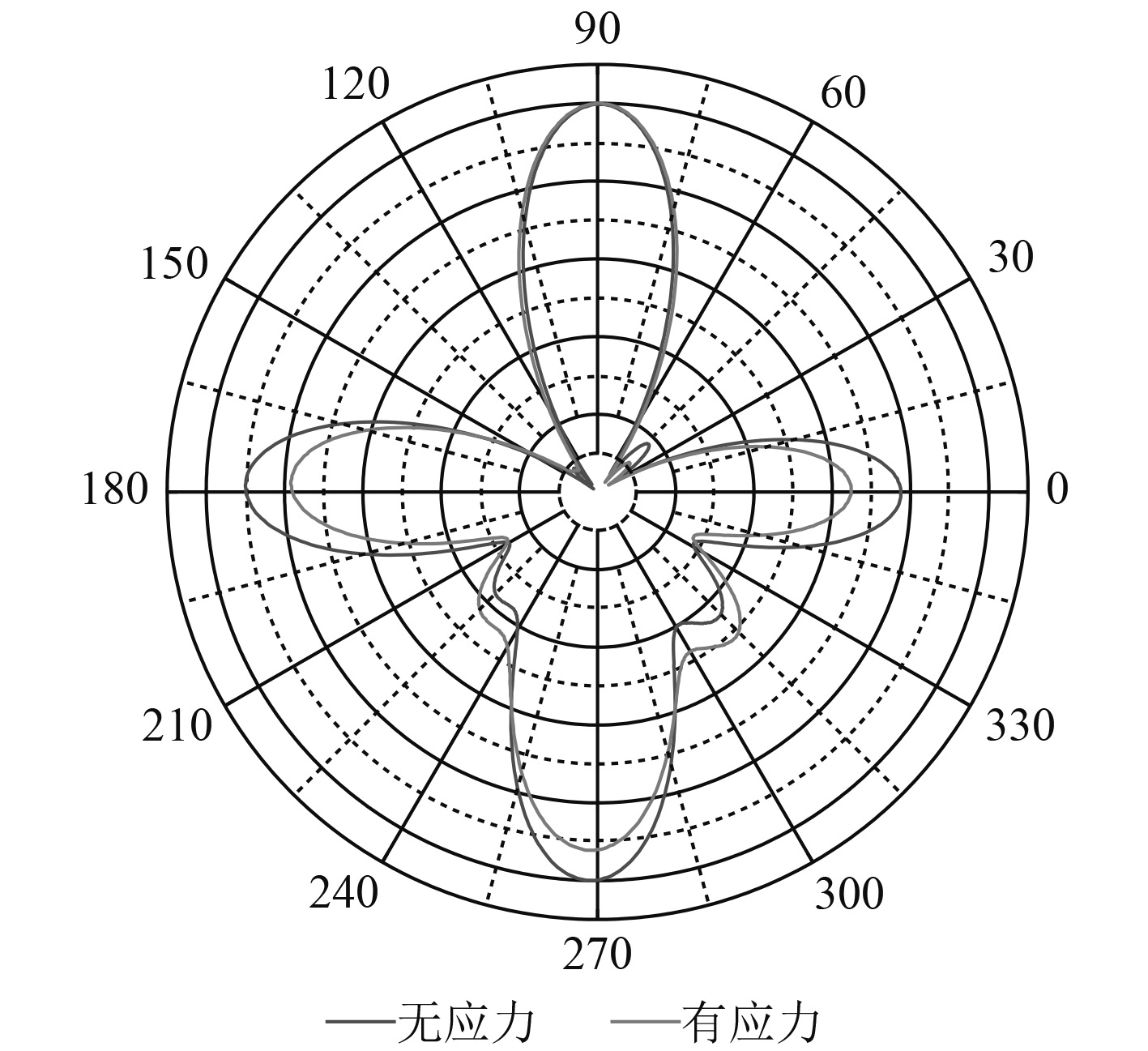图 4 结构声辐射指向性对比 Fig. 4 Comparison of structural acoustic radiation directivity
3.2 焊接残余应力幅值对结构声辐射的影响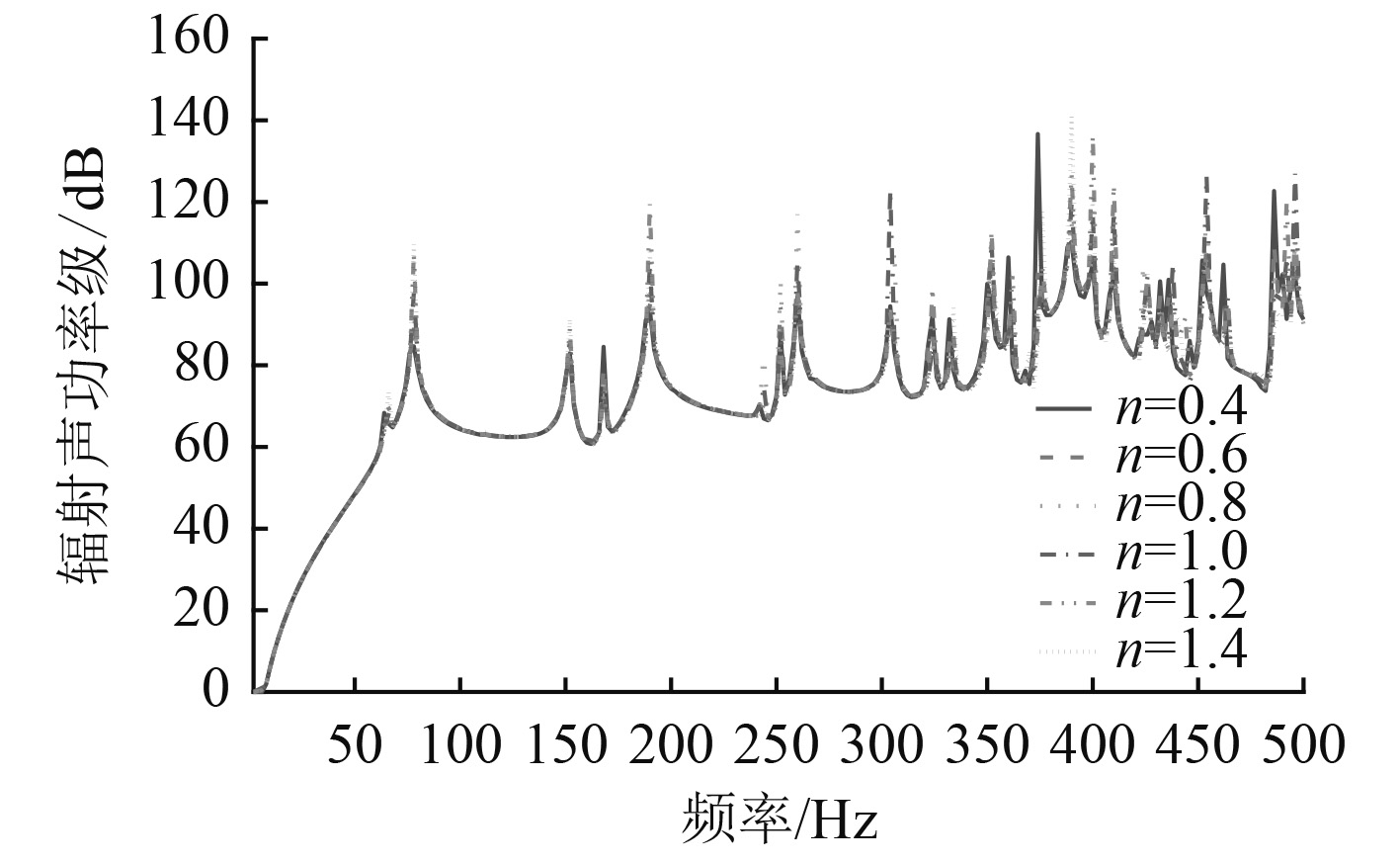图 5 不同应力幅值下结构声辐射功率 Fig. 5 Structural acoustic radiation power of different stress amplitude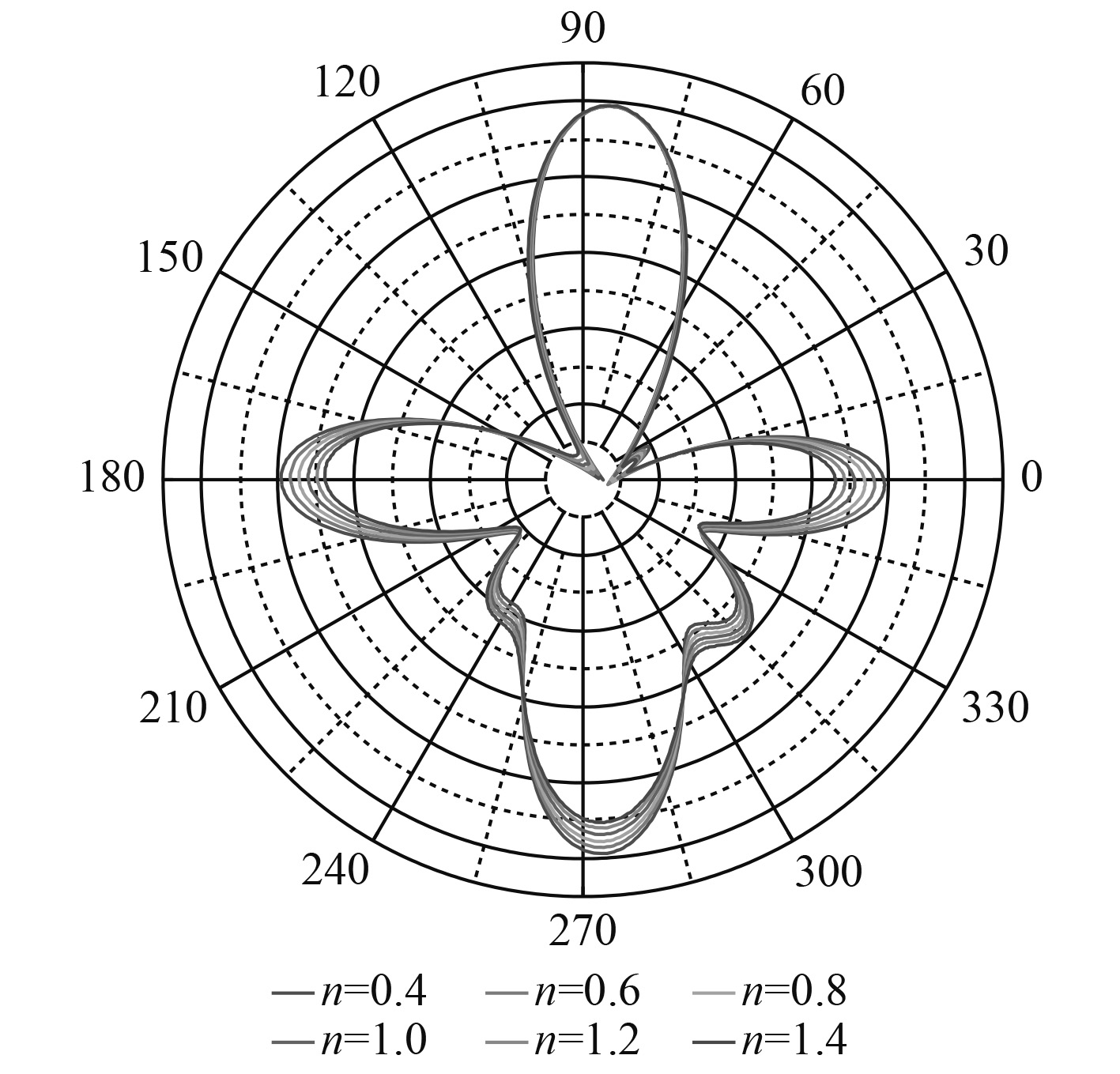图 6 不同应力幅值下结构声辐射指向性 Fig. 6 Structural acoustic radiation directivity of different stress amplitude
3.3 焊缝类型的影响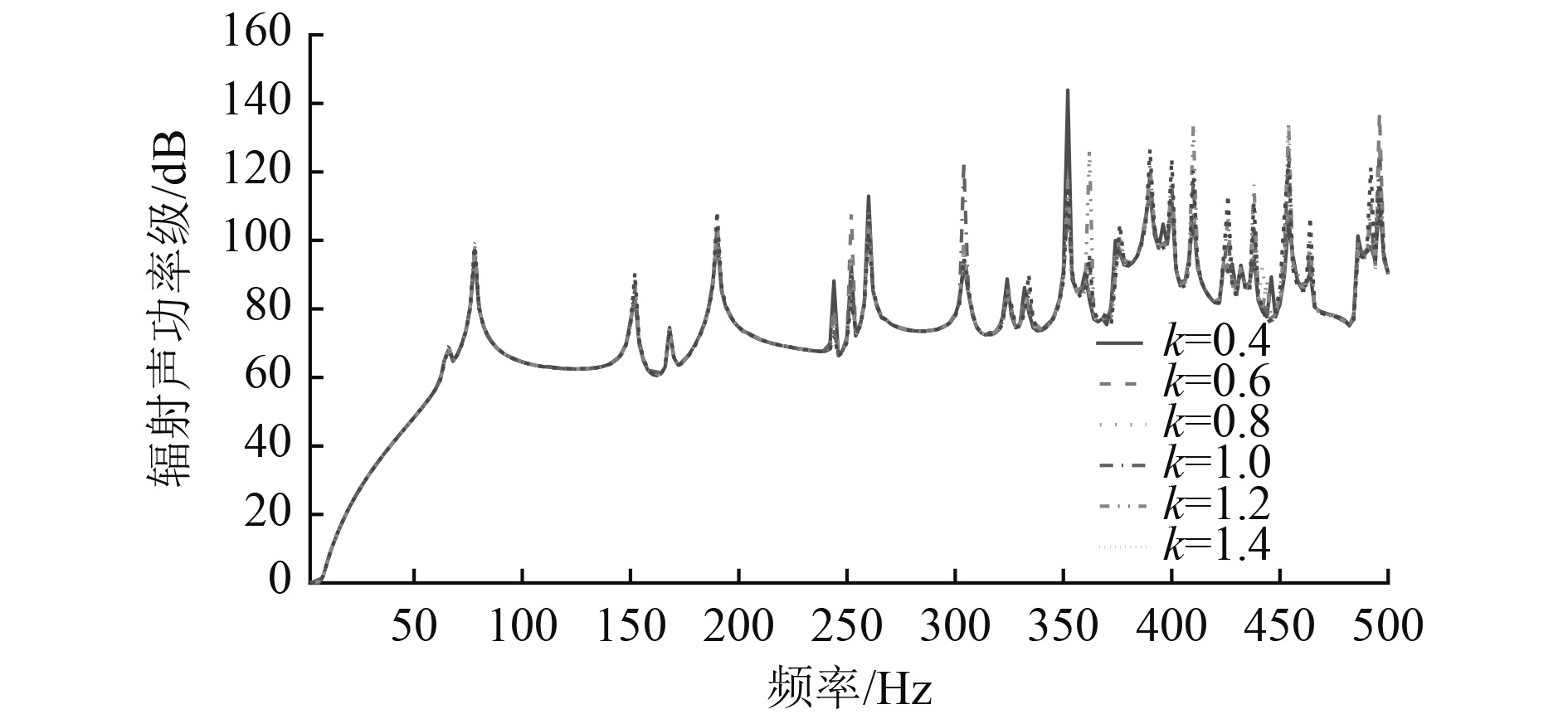图 7 不同环向焊缝应力下结构声辐射功率 Fig. 7 Structural acoustic radiation power of different stress of circumferential weld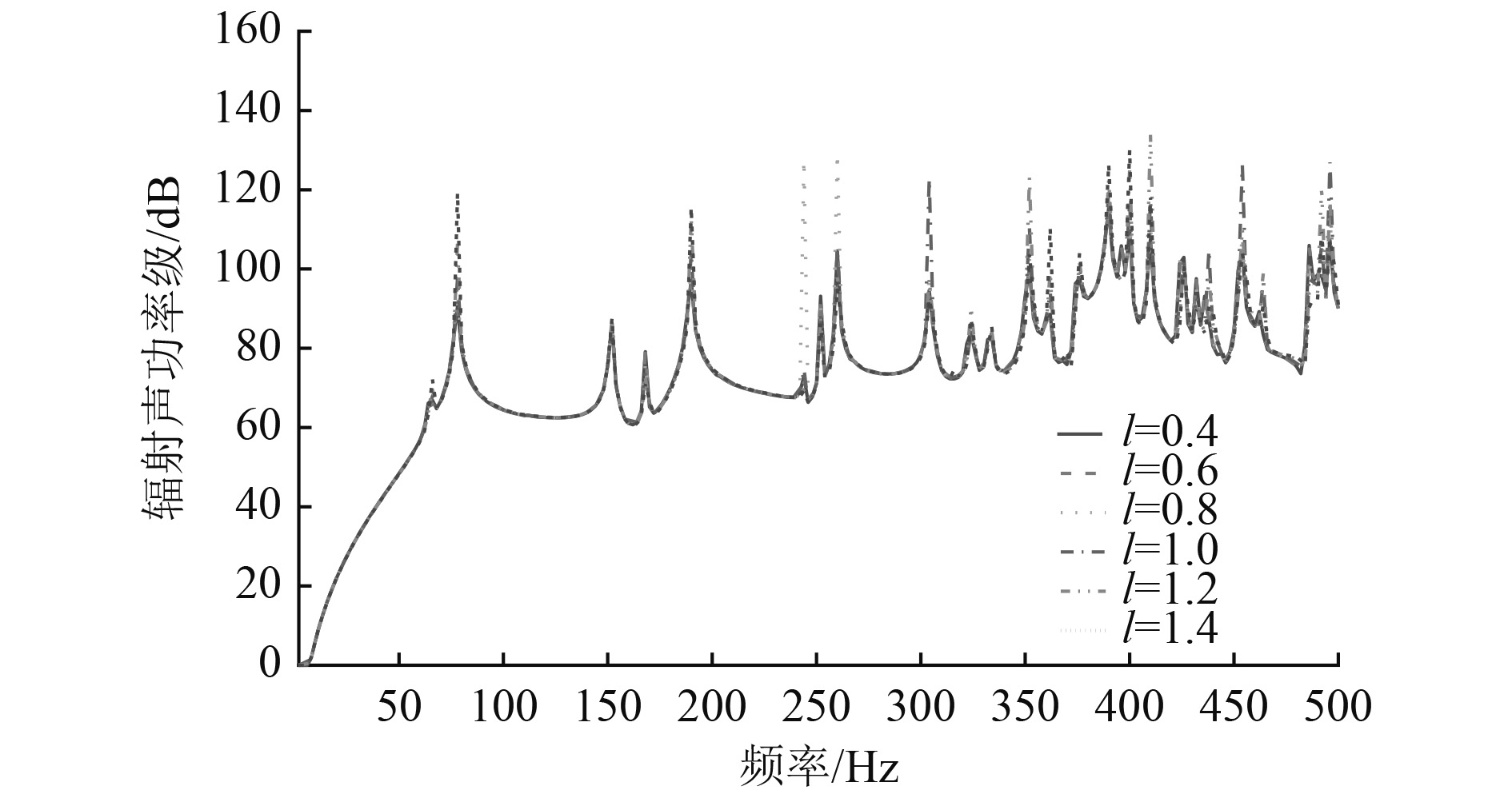图 8 不同轴向焊缝应力下结构声辐射功率 Fig. 8 Structural acoustic radiation power of different stress of axial weld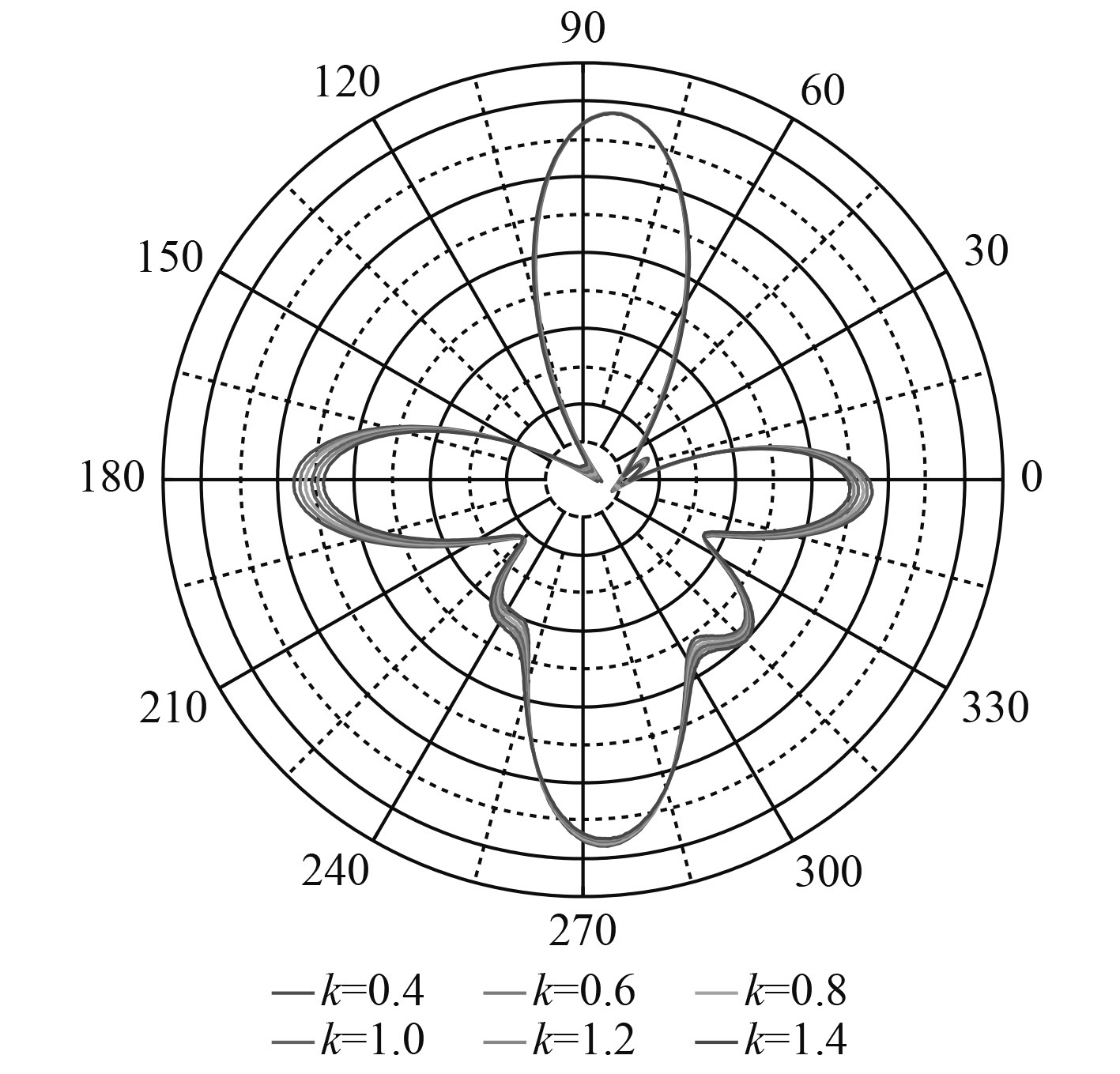图 9 不同环向焊缝应力下声辐射指向性 Fig. 9 Structural acoustic radiation directivity of different stress of circumferential weld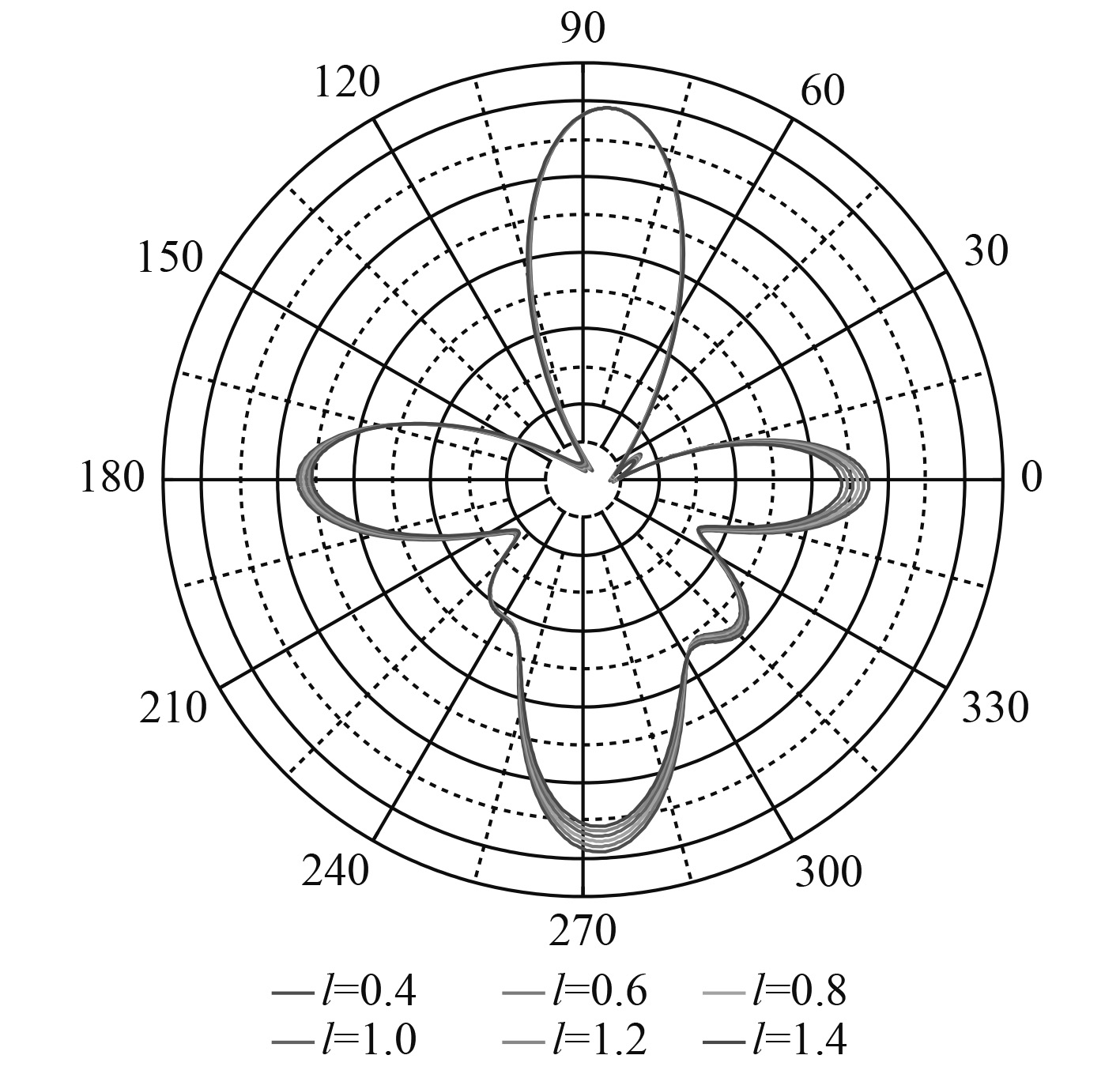图 10 不同轴向焊缝应力下声辐射指向性 Fig. 10 Structural acoustic radiation directivity of different stress of axial weld
4 结　语

1）焊接残余应力的存在改变了结构的刚度，继而改变了结构的动力学特性，影响了结构的声辐射特性。

2）焊接残余应力的幅值的变化主要影响结构最大声辐射功率值，并且会使声辐射功率极值的频率产生偏移。各频率辐射声功率极值会出现在不同的幅值参数取值时。焊接残余应力幅值的变化会对结构声辐射指向性产生一定的影响。

3）在低频段，环向焊缝应力大小对结构声辐射功率影响较小。在高频段，环向焊缝应力大小的变化改变了结构声辐射功率极值。轴向焊缝应力大小的变化会对结构声辐射功率的极值和最大值产生影响。环向和轴向焊缝应力大小的变化对结构声辐射指向性的影响较小。

  缪宇跃, 李天匀, 朱翔, 等. 浅海中圆柱壳的声辐射特性分析[J]. 哈尔滨工程大学学报, 2017, 38(5): 719-726. MIAO Yu-yue, LI Tian-yun, ZHU Xiang, et al. Research on the acoustical radiation characteristics of cylindrical shells in ashallow sea[J]. Journal of Harbin Engineering University, 2017, 38(5): 719-726.  陈清坤, 陈美霞, 和卫平, 等. 舱壁与压载对流场中有限长圆柱壳声辐射影响[J]. 舰船科学技术, 2010, 32(11): 21-25. CHEN Qing-kun, CHEN Mei-xia, HE Wei-ping, et al. Impact of bulkhead and ballast on acoustic radiation of submerged finite cylindrical shell[J]. Ship Science and Technology, 2010, 32(11): 21-25.  张聪, 陈美霞, 高菊, 等. 双层加筋圆柱壳在不同舷间连接形式下的声辐射性能分析[J]. 固体力学学报, 2011, 32(5): 465-474. ZHANG Cong, CHEN Mei-xia, GAO Ju, et al. Analysis of sound radiation of double stiffened shells connected by different structures[J]. Chinese Journal of Solid Mechanics, 2011, 32(5): 465-474.  LOOCK J V D, DÉCULTOT D, LÉON F, et al. Acoustic radiation of a submerged cylindrical shell in low frequency[J]. Journal of the Acoustical Society of America, 2013, 133(1): EL26. DOI:10.1121/1.4769398  GUO W, LI T, ZHU X, et al. Vibration and acoustic radiation of a finite cylindrical shell submerged at finite depth from the free surface[J]. Journal of Sound and Vibration, 2017, 393: 338-352. DOI:10.1016/j.jsv.2017.01.003  邓博文, 朱翔, 李天匀, 等. 不同模型及不同载荷形式对单层圆柱壳声振特性的影响分析[J]. 舰船科学技术, 2015, 37(12): 13-17. DENG Bo-wen, ZHU Xiang, LI Tian-yun, et al. Effects of different models and driving forces on vibroacoustic characteristic of single cylinder shell[J]. Ship Science and Technology, 2015, 37(12): 13-17. DOI:10.3404/j.issn.1672-7649.2015.12.003  陈美霞, 骆东平, 王祖华, 等. 激励力对双层圆柱壳声辐射性能的影响[J]. 船舶力学, 2005, 9(2): 124-130. CHEN Mei-xia, LUO Dong-ping, WANG Zu-hua, et al. Effect of driving forces property on characteristics of sound radiation from finite double cylindrical shell[J]. Journal of Ship Mechanics, 2005, 9(2): 124-130. DOI:10.3969/j.issn.1007-7294.2005.02.018  刘佩, 刘书文, 黎胜. 潜深对水下圆柱壳振动声辐射特性的影响[J]. 舰船科学技术, 2014, 36(5): 36-41. LIU Pei, LIU Shu-wen, LI Sheng. The effect of immersion depth of submerged cylindrical shell on vibro-acoustic characteristics[J]. Ship Science and Technology, 2014, 36(5): 36-41. DOI:10.3404/j.issn.1672-7649.2014.05.008  FARSHIDIANFAR A, OLIAZADEH P. Free vibration analysis of circular cylindrical shells: comparison of different shell theories[J]. International Journal of Mechanics and Applications, 2012, 2(5): 74-80. DOI:10.5923/j.mechanics.20120205.04  ZHAO X, GENG Q, LI Y. Vibration and acoustic response of an orthotropic composite laminated plate in a hygroscopic environment[J]. Journal of the Acoustical Society of America, 2013, 133(3): 1433-42. DOI:10.1121/1.4790353  李良碧, 万正权, 王自力, 等. 环肋圆柱壳结构焊接残余应力和变形的数值模拟[J]. 焊接技术, 2011, 40(3): 9-12. LI Liang, WAN Zheng-quan, WANG Zi-li, et al. Numerical simulation on welding residual stresses and deformation of ring-stiffened circular cylindrical shell[J]. Welding Technology, 2011, 40(3): 9-12. DOI:10.3969/j.issn.1002-025X.2011.03.003  LIU Y, CHEN L. The effect of weld residual stress on the free vibrational characteristics of cylindrical shell through the analytical method[J]. Journal of Vibroengineering, 2016, 18(1): 334-349.  YANG N, CHEN L, YI H, et al. The effect of welding residual stress on the free vibration of underwater cylindrical shell[J]. Journal of Vibroengineering, 2016, 18(4).  陈炉云, 李磊鑫, 张裕芳. 含局部预应力的圆柱壳结构声辐射特性分析[J]. 上海交通大学学报, 2014, 48(8): 1084-1089. CHEN Lu-yun, LI Lei-xin, ZHANG Yu-fang. Characteristics analysis of structural-acoustic of cylindrical shell with prestress in local areas[J]. Journal of Shanghai Jiao Tong University, 2014, 48(8): 1084-1089.  洪江波, 杜仲民, 侯海量, 等. 大型耐压壳环焊缝焊接残余应力实验研究[J]. 船舶工程, 2006, 28(5): 14-18. HONG Jiang-bo, DU Zhong-min, HOU Hai-liang. Experimental study of residual stress in girth weld of large pressure hull[J]. Ship Engineering, 2006, 28(5): 14-18. DOI:10.3969/j.issn.1000-6982.2006.05.016